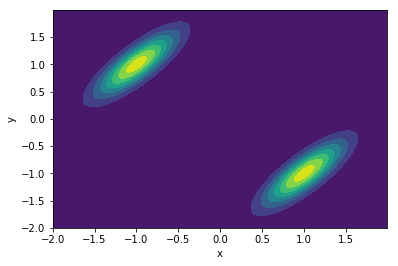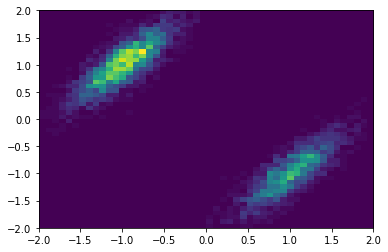# Abstract

This article provides the recipes, simple Python codes and mathematical proof to the most basic form of Gibbs sampling.

# What is Gibbs sampling?

Gibbs sampling is a method to generate samples from a multivariant distribution $P(x_1, x_2, …, x_d)$ using only conditional distributions $P(x_1|x_2…x_d)$, $P(x_2|x_1, x_3…x_d)$ and so on. It is used when the original distribution is hard to calculate but the conditional distributions are available.

# How does it work?

## Procedure

Starting with a sample $\mathbf{x}=(x_1, x_2…x_d)$, to generate a new sample $\mathbf{y}=(y_1, y_2…y_d)$, do the following

1. Sample $y_1$ from $P(y_1\vert x_2, x_3…x_d)$.
2. Sample $y_2$ from $P(y_2\vert y_1, x_3…x_d)$.
3. Sample $y_3$ from $P(y_3\vert y_1, y_2, x_4…x_d)$
4. … and so on. The last term is sampling $y_d$ from $P(y_d\vert y_1, y_2…y_{d-1})$.
5. Now we have the new sample $\mathbf{y}$. Repeat for the next sample.

Note that the value of a new sample depends only on the the previous one, and not the one before, Gibbs sampling belongs to a class of methods called Markov Chain Monte Carlo (MCMC).

## Example

Consider a mixture of two 2-dimensional normal distribution below with joint distribution $P(x,y) = \frac{1}{2} \left( \mathcal{N}(\mu_1, \text{cov}_1) + \mathcal{N}(\mu_2, \text{cov}_2) \right)$.We can use the procedure above to to generate samples using only the conditional distribution $P(x\vert y)$ and $P(y\vert x)$. The sample chain could initially be trapped in one local distribution:But if enough number of points are sampled, the original distribution is recovered:The codes that generate this example can be found here.

# Why does it work?

This section outlines the proof of Gibbs sampling. I mostly follow MIT 14.384 Time Series Analysis, Lectures 25 and 26.

## Markov Chain Monte Carlo (MCMC)

Gibbs sampling generates a Markov chain. That’s obvious because the series $X^{(1)}$, $X^{(2)}$, $X^{(3)}$ … depends only on the previous value. An important concept to understand is the transition Probability $P(\mathbf{x}, \mathbf{y})$, the probability of generating sample $\mathbf{y}$ from the previous sample $\mathbf{x}$.

Suppose we want to sample a distribution $\pi(\mathbf{x})$, we want to generate samples in a way that the new sample still follows distribution $\pi(\mathbf{x})$. Mathematically, for transition probability $P(\mathbf{x}, \mathbf{y})$, the probability measure $\pi(\mathbf{x})$ is invariant if

$\begin{equation} \pi(\mathbf{y})=\int P(\mathbf{x}, \mathbf{y}) \pi(\mathbf{x}) d \mathbf{x} \end{equation}$

and the transition probability is

1. irreducible (i.e. any point can reach any other points)
2. aperiodic

The MCMC problem is to find a transition probability $P(\mathbf{x}, \mathbf{y})$ such that $\pi(\mathbf{x})$ is invariant.

## Proof

The procedure of Gibbs sampling outlined previously can be written as a transition probability

\begin{align} P(\mathbf{x},\mathbf{y}) &= \pi(y_1|x_2,x_3,..,x_d) \pi(y_2|y_1,x_3,..,x_d)...\pi(y_d|y_1,y_2,..,y_{d-1}) \\ &=\prod_k \pi(y_k|y_1...y_{k-1}, x_{k+1}...x_{d}) \end{align}

Let $\pi(\mathbf{x})$ be the distribution we want to sample from. We need to show $\pi(\mathbf{x})$ is invariant under this transition, i.e.

\begin{align} \int P(\mathbf{x,y}) \pi(\mathbf{x}) d\mathbf{x} = \pi(\mathbf{y}) \label{trans} \end{align}

In other words, if we follow $P(\mathbf{x},\mathbf{y})$ to get new sample $\mathbf{y}$ based on the previous sample $\mathbf{x}$, and repeat this process over and over again, we stay in the same distribution $\pi(\mathbf{x})$.

Since we need to integrate over $\mathbf{x}$, the first thing is to rewrite $P(\mathbf{x},\mathbf{y})$ using Bayes rule so that there’s no conditional on $\mathbf{x}$. Bayes rule is

$P(A|B) P(B) = P(B |A) P(A)$

or if there’s always a conditional on $C$:

$P(A|B,C) P(B|C) = P(B |A,C) P(A|C)$

Using

\begin{align} A&=y_k \\ B&= x_{k+1},...,x_{d}\\ C&=y_1,..,y_{k-1} \end{align}

We get

\begin{align} \pi(y_k|y_1...y_{k-1}, x_{k+1}...x_{d}) = \frac{\pi(x_{k+1},...,x_{d}|y_1,..,y_{k} )\pi(y_k|y_1,..,y_{k-1} )}{\pi(x_{k+1},...,x_{d}|y_1,..,y_{k-1})} \end{align}

Substituting this to Eq.(\ref{trans}), and noticing that $\pi(y_k\vert y_1,..,y_{k-1} )$ does not depend on the integrating variable $\mathbf{x}$,

\begin{align} \int P(\mathbf{x,y}) \pi(\mathbf{x}) d\mathbf{x} = \prod_k\pi(y_k|y_1,..,y_{k-1} ) \int \prod_k \frac{\pi(x_{k+1},...,x_{d}|y_1,..,y_{k} )}{\pi(x_{k+1},...,x_{d}|y_1,..,y_{k-1})} \pi(\mathbf{x}) d\mathbf{x} \end{align}

The first product

\begin{align} \prod_k\pi(y_k|y_1,..,y_{k-1} ) = \pi(\mathbf{y}) \end{align}

because

\begin{align} \pi(\mathbf{y}) &= \pi(y_1, y_2,..,y_d)\\ &= \pi(y_d|y_1,..,y_{d-1})\pi(y_1,..,y_{d-1})\\ &= \pi(y_d|y_1,..,y_{d-1})\pi(y_{d-1}|y_1,..,y_{d-2})\pi(y_1,..,y_{d-2}) \\ &\text{... and so on} \end{align}

So we have

\begin{align} \int P(\mathbf{x,y}) \pi(\mathbf{x}) d\mathbf{x} = \pi(\mathbf{y}) \int \prod_k \frac{\pi(x_{k+1},...,x_{d}|y_1,..,y_{k} )}{\pi(x_{k+1},...,x_{d}|y_1,..,y_{k-1})} \pi(\mathbf{x}) d\mathbf{x} \end{align}

We need to show the integral on the right hand side to be exactly 1.

We start by writing out the terms explicitly and use $\pi(x_1,..x_d) = \pi(x_1\vert x_2…x_d)\pi(x_2…x_d)$,

\require{cancel} \begin{align} \int \prod_k \frac{\pi(x_{k+1},...,x_{d}|y_1,..,y_{k} )}{\pi(x_{k+1},...,x_{d}|y_1,..,y_{k-1})} \pi(\mathbf{x}) d\mathbf{x} = \int \frac{\pi(x_2...x_d|y_1)}{\cancel{\pi(x_2...x_d)}} \frac{\pi(x_3...x_d|y_1,y_2)}{\pi(x_3...x_d|y_1)} ... \pi(x_1|x_2...x_d)\cancel{\pi(x_2...x_d)} dx_1 dx_2...dx_d \end{align}

The only term that depends on $x_1$ is $\pi(x_1\vert x_2…x_d)$ and it is integrated out to be 1. The integral becomes

\begin{align} = \int \frac{\pi(x_2...x_d|y_1)}{\pi(x_3...x_d|y_1)} \frac{\pi(x_3...x_d|y_1,y_2)}{\pi(x_4...x_d|y_1,y_2)} ... \pi(x_d|y_1...y_{d-1}) dx_2...dx_d \end{align}

Turning each fraction into a conditional probability

\begin{align} \frac{\pi(x_2...x_d|y_1)}{\pi(x_3...x_d|y_1)} = \pi(x_2|x_3..x_d,y_1) \end{align}

and so on. The integral becomes

\begin{align} = \int \pi(x_2|x_3..x_d,y_1) \pi(x_3|x_4..x_d,y_1, y_2) ... \pi(x_d|y_1...y_{d-1}) dx_2...dx_d \end{align}

Only the first term depends on $x_2$ and it is integrated out to be 1. After that, only the leading term depends on $x_3$ and it is integrated out to be 1 and so on. Therefore the whole integral is 1 resulting in

\begin{align} \int P(\mathbf{x,y}) \pi(\mathbf{x}) d\mathbf{x} = \pi(\mathbf{y}) \end{align}

So the transition probability from Gibbs sampling is invariant. If we sample according to the Gibbs scheme, we can get unbiased samples of $\pi(\mathbf{x})$.